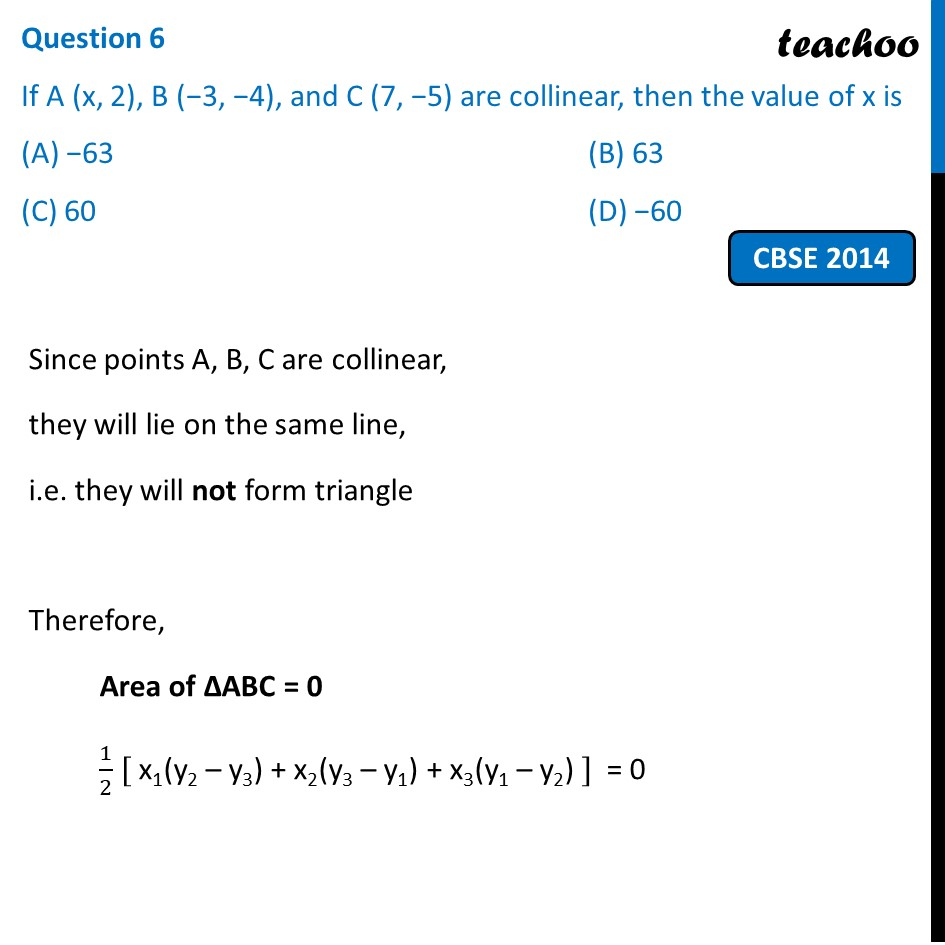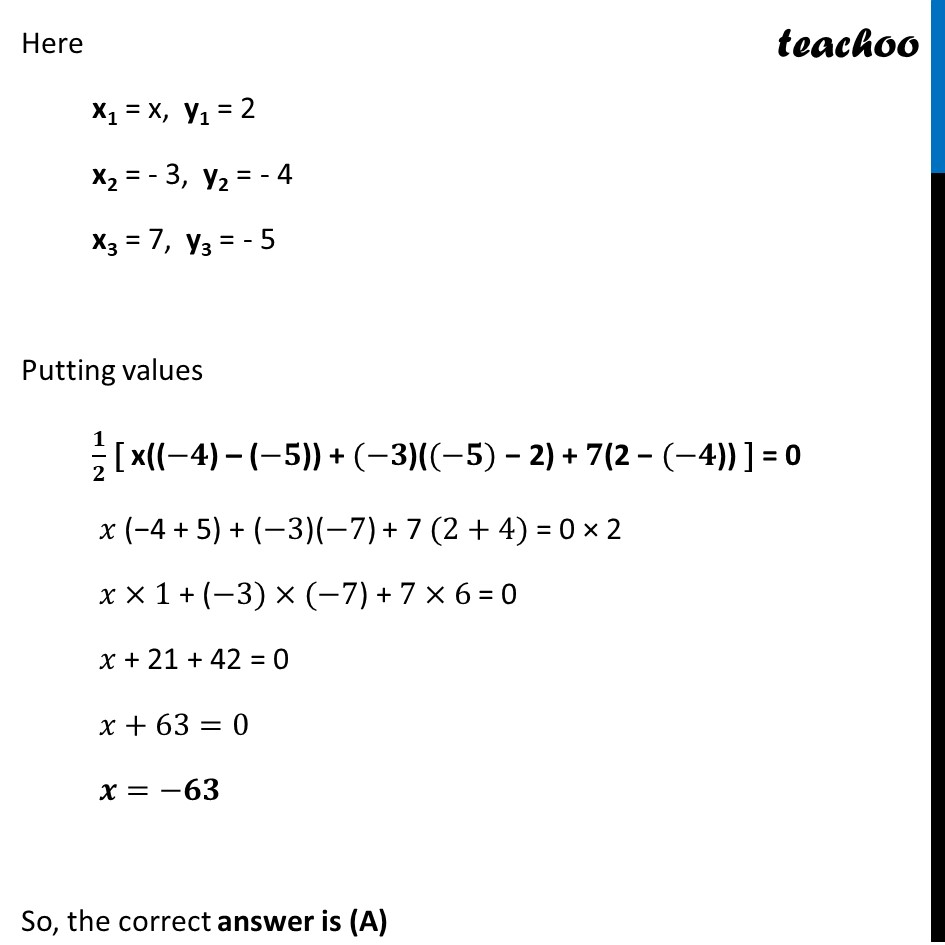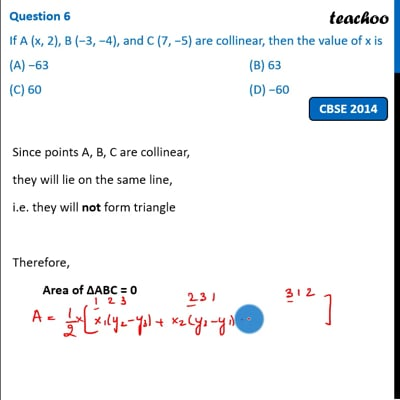Past Year MCQ

Chapter 7 Class 10 Coordinate Geometry (Term 1)
Serial order wise

## (D) −60

This question is similar to Ex 7.3, 2 (i) - Chapter 7 Class 10 Coordinate GeometryThis video is only available for Teachoo black users

### Transcript

Question 6 If A (x, 2), B (−3, −4), and C (7, −5) are collinear, then the value of x is (A) −63 (B) 63 (C) 60 (D) −60 Since points A, B, C are collinear, they will lie on the same line, i.e. they will not form triangle Therefore, Area of ∆ABC = 0 1/2 [ x1(y2 – y3) + x2(y3 – y1) + x3(y1 – y2) ] = 0 Here x1 = x, y1 = 2 x2 = - 3, y2 = - 4 x3 = 7, y3 = - 5 Putting values 𝟏/𝟐 [ x((−𝟒) – (−𝟓)) + (−𝟑)((−𝟓) − 2) + 𝟕(2 − (−𝟒)) ] = 0 𝑥 (−4 + 5) + (−3)(−7) + 7 (2+4) = 0 × 2 𝑥×1 + (−3)×(−7) + 7×6 = 0 𝑥 + 21 + 42 = 0 𝑥+63=0 𝒙=−𝟔𝟑 So, the correct answer is (A)